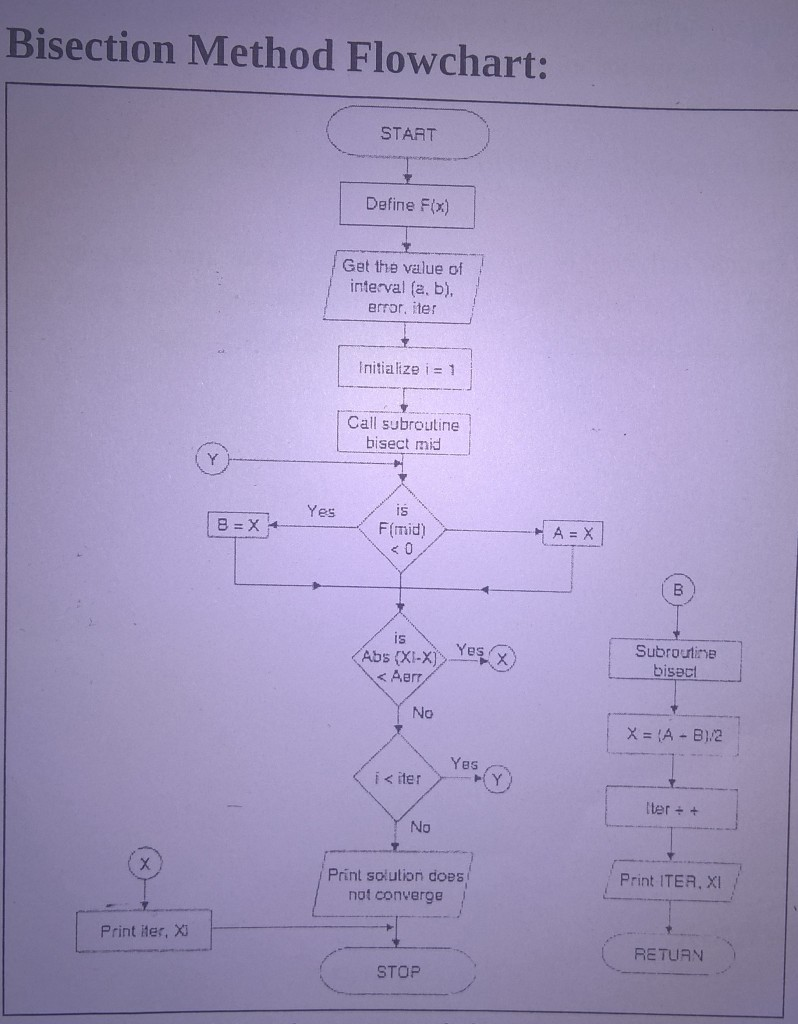Algorithm Bisection Method

Algorithm:

1. Start

2. Read x1, x2, e
*Here x1 and x2 are initial guesses
e is the absolute error i.e. the desired degree of accuracy*

3. Compute: f1= f(x1) and f2=f(x2)

4. If (f1*f2) <0, then display initial guesses are wrong and goto 11
Otherwise continue.

5. x=(x1+x2)/2

6. If |(x1-x2)/x|<e), then display x and goto (11)
*Here || refers to the modulus*

7.  Else, f=f(x)

8. If ((f*f1)>0, then x1=x and f1=f

9. Else, x2=x and f2=f

10. Goto 5
* Now the loop continues with new values*

11. Stop

Flow Chart: